MOBILE MATH – Math on the Go
www.mobilemath.ca

# 1 - Numeracy - Adding and Subtracting

Summary – Examples Covered

Screen Shot

1.1  – Numeracy – Adding Small Positive Numbers

Learning Goal:

By the end of this lesson I should be able to ADD single and two digit positive numbers together WITHOUT the use of a calculator (technology).

Techniques:  Picture Model,            Number Model

Examples:

(a)            7 + 9

(b)           4 + 7 + 6

(c)            17 + 9

(d)           14 + 19 + 3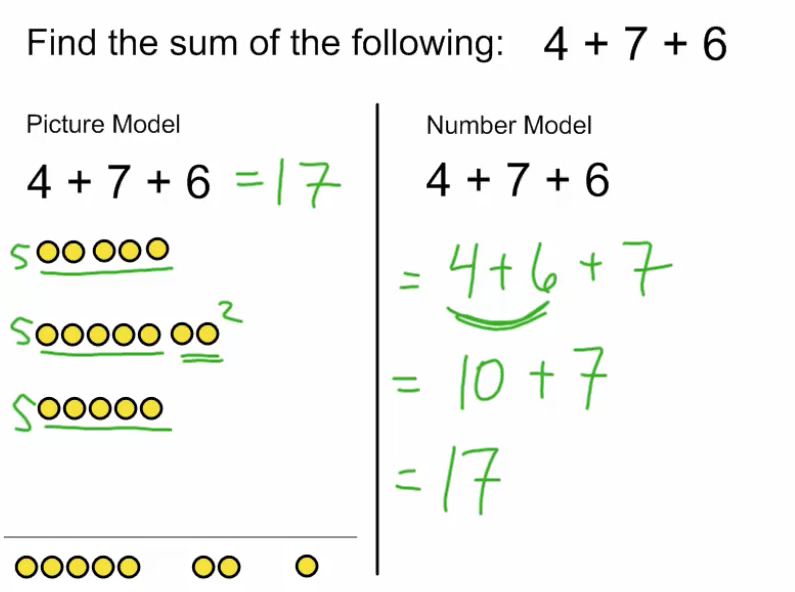1.2  – Numeracy – Adding Larger Positive Numbers

Learning Goal:

By the end of this lesson I should be able to ADD double and triple digit positive numbers together WITHOUT the use of a calculator (technology).

Techniques:  Number Model,          Column Model

Examples:

(a)            59 + 27

(b)           146 + 82

(c)            522 + 394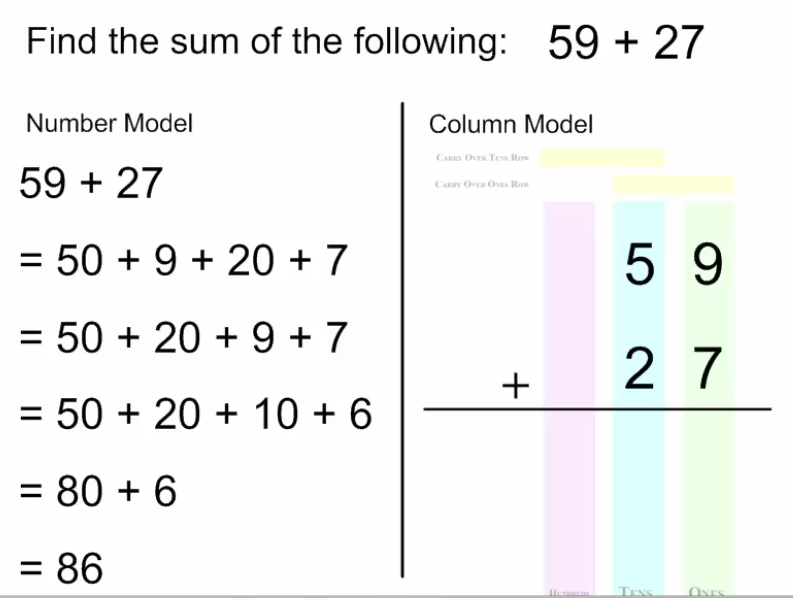1.3  – Numeracy – Adding Decimals

Learning Goal:

By the end of this lesson I should be able to ADD positive numbers together that have one or two decimal places WITHOUT the use of a calculator (technology).

Techniques:  Number Model,          Column Model

Examples:

(a)            3.5 + 2.4

(b)           5.45 + 1.73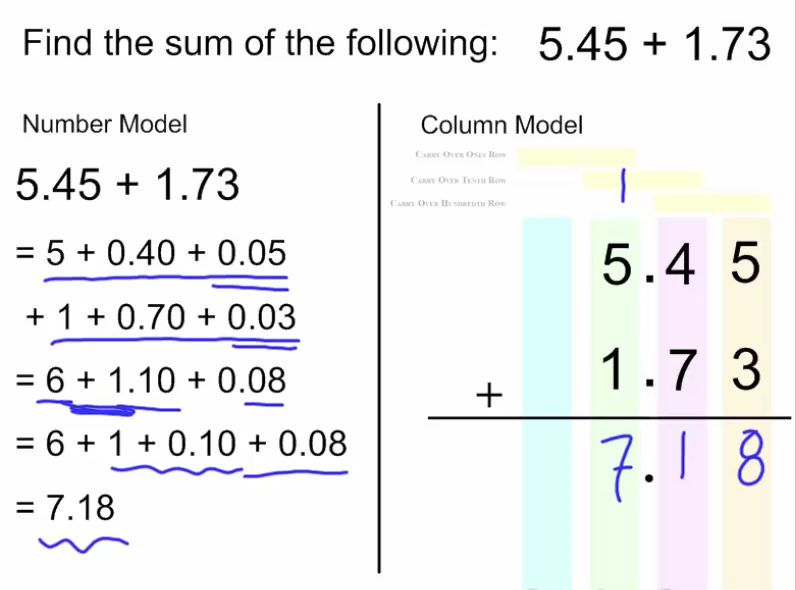1.4  – Numeracy – Adding Positive and Negative Numbers

(Zero Principle)

Learning Goal:

By the end of this lesson I should be able to ADD positive and negative numbers together WITHOUT the use of a calculator (technology).

Techniques:  Integer Chip Model, Modified Integer Chip Model

Examples:

(a)           3 + 2

(b)            6 + (-4)

(c)            3 + (-4)

(d)            (-3) + (-2) + 1

(e)           4 + (-4) + (-2)

(f)             (-13) + (15)

(g)             55 + (-48)1.5  – Numeracy – Subtracting Positive Numbers

Learning Goal:

By the end of this lesson I should be able to SUBTRACT positive numbers from other numbers (positive or negative) WITHOUT the use of a calculator (technology).  Part 1

Techniques:  Integer Chip Model

Examples:

(a)            6 - 4

(b)           (-4) - 2

(c)            5 + (-2)  vs  5 - 2

(d)           (-3) – 2 - 1  vs  (-3) + (-2) + (-1)1.6  – Numeracy – Subtracting Negative Numbers

Learning Goal:

By the end of this lesson I should be able to SUBTRACT negative numbers from other numbers (positive or negative) WITHOUT the use of a calculator (technology).  Part 2

Techniques:  Integer Chip Model

Examples:

(a)            (-3) - 4

(b)           2 – (-3)

(c)            5 + 2   vs  5 – (-2)

(d)           (-3) – (-2) – (-1)   vs   (-3) + 2 + 1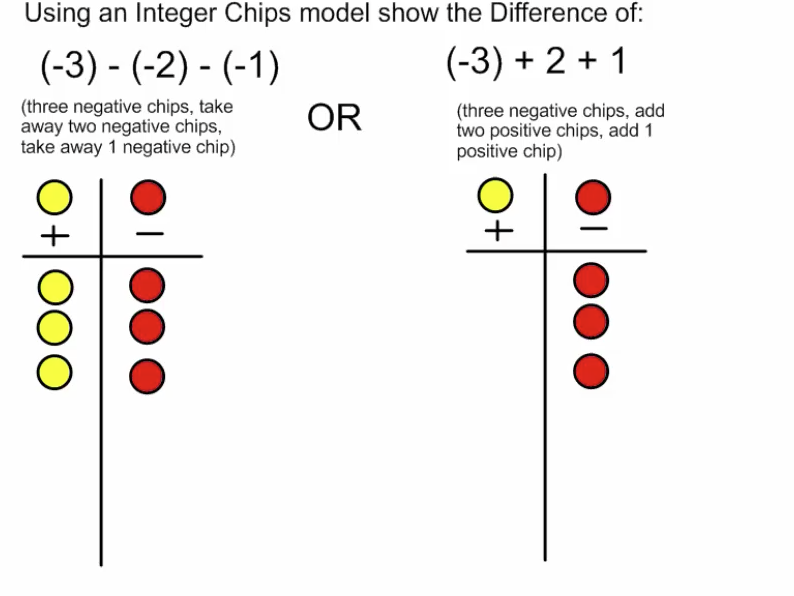1.7 – Numeracy – Subtracting Positive Numbers (column)

Learning Goal:

By the end of this lesson I should be able to SUBTRACT positive numbers using a Column Model, WITHOUT the use of a calculator (technology).

Techniques:  Number Model,          Column Model

Examples:

(a)            35 - 24

(b)           22 - 7

(c)            45 - 28

(d)           742 - 387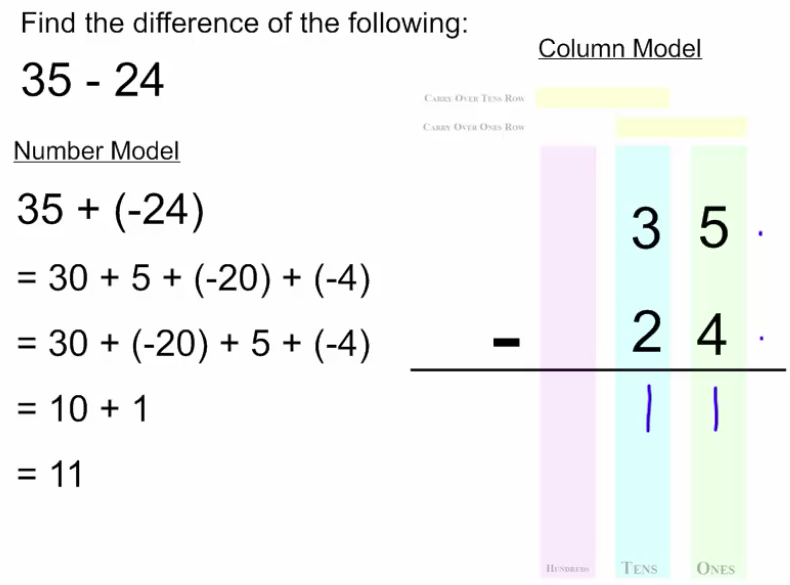1.8  – Numeracy – Mixed Up

Learning Goal:

By the end of this lesson I should be able to EVALUATE mixed addition and subtraction problems WITHOUT the use of a calculator (technology).

Techniques:  Integer Chip Model,   Number Model

Examples:

(a)            (-13) - 14

(b)           24 - (-11) + (-7)

1.9  – Numeracy – Final Assessment

Task 1.9a - Review - Word

Task 1.9a - Review - Pdf

Final Assessment 1.9b - Word

Final Assessment 1.9b - Pdf

Summative Activites for the Adding/Subtracting Unit